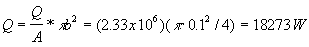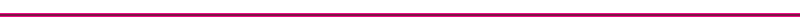Piston Heat Transfer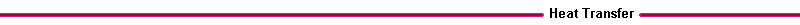Figure 16.  Piston Heat Transfer

The piston crown conducts heat from the conduction gases to the cooling oil, to the piston rings, and the piston skirt. Frictional heating is also a source of heat.

Engine Heat Transfer CorrelationsFor the overall average heat transfer from the gas to the cylinder coolant, convection type heat transfer equations are used.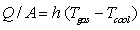Where:

Q = overall heat transfer (W/m2)
A = reference cylinder area (m2)
Tgas = effective gas temperature, typically 800 C
Tcool = coolant temperature, typically 80 C
h= heat transfer coefficient

The heat transfer coefficient depends on the engine geometric parameters, such as the exposed cylinder area and bore, and piston speed. The coefficient varies with location and piston position. The coefficient is found from a Nusselt - Reynolds number correlation.

Nusselt # = a (Reynolds #)m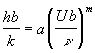Where:

b = cylinder characteristic length (m), usually chosen to be the cylinder bore.
k = gas thermal conductivity, (W/mK), typical value 0.06
v = gas thermal diffusivity, (m2/s), typical value 100 x 10-6
U = characteristic gas velocity (m/s)

Depending on the correlation used, the characteristic gas velocity U is the mean piston speed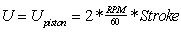the average intake velocity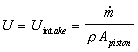or a combustion velocity. The coefficient a and exponent m are found from engine experiments. There are three types of heat transfer coefficients used in the computation of engines heat transfer.

Table III. Types of Heat Transfer Coefficients
 Averaging Used for h(x,t) averaged over time & space overall steady state energy balance calculations h(x,t) instantaneous, space average heat transfer versus crank angle h(x,t) instantaneous, local local calculations of thermal stress

The peak values of the instantaneous and local coefficients can be many times higher than the averaged values.

A frequently used correlation for an average h is that of Taylor (ref), which uses the mean piston speed for the characteristic velocity.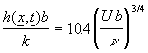For a 1000 rpm engine with a 0.1m stroke, combustion gas temperature of 1000C, and coolant temperature of 80 C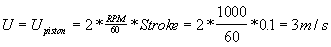Where:

b = 0.1 m
k = 0.06 W/mK
v = 100 x 10 -6 m2/s

the average heat transfer coefficient isThe average heat transfer per unit area from the cylinder to the coolant isFor a reference cylinder area of p b2/4, the average heat transfer is